Quick Search for:  in language:
Code/Articles  |  Newest/Best  |  Community  |  Jobs  |  Other  |  Goto  |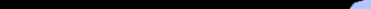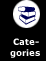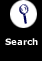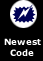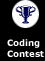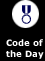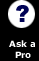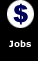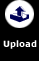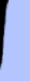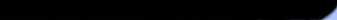Perl Stats

Code: 74,273. lines
Jobs: 24. postings

How to support the site

 You are in:***Site Home***.NetASP / VbScriptC / C++Cold FusionDelphiJava / JavascriptLISPPerlPHPSQLVisual Basic  Does your code think in ink?

Latest Code Ticker for Perl.CGIScripterBy David Simpson on 11/24(Screen Shot)CalenderBy Jeff Mills on 11/20quikpollBy Jeff Mills on 11/20Encrypt PasswordBy Jeff Mills on 11/20Rock, Paper, Scissors w/ GUIBy Kurt Rudolph on 11/19

Daily Code Email

 Affiliate Sites

simplae maths program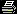Print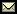Email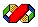Submitted on: 10/15/2003 2:24:11 PM By: Harry Wright  Level: BeginnerUser Rating: Unrated Compatibility:5.0 (all versions)Users have accessed this code 481 times. (About the author)

it works out sums like add divide subtract sqaure and double

Can't Copy and Paste this?
```
=**************************************
= for :simplae maths program
=**************************************
nope```
code:
Can't Copy and Paste this?
 Terms of Agreement:    By using this code, you agree to the following terms...    1) You may use this code in your own programs (and may compile it into a program and distribute it in compiled format for languages that allow it) freely and with no charge.    2) You MAY NOT redistribute this code (for example to a web site) without written permission from the original author. Failure to do so is a violation of copyright laws.    3) You may link to this code from another website, but ONLY if it is not wrapped in a frame.  4) You will abide by any additional copyright restrictions which the author may have placed in the code or code's description. ``` =************************************** = Name: simplae maths program = Description:it works out sums like add = divide subtract sqaure and double = By: Harry Wright = = Inputs:just numbers = = Returns:answers = = Assumes:it has to be run in shell or d = os = =This code is copyrighted and has = limited warranties.Please see http://w = ww.Planet-Source-Code.com/vb/scripts/Sho = wCode.asp?txtCodeId=547&lngWId;=6 =for details. =************************************** #include int square(int); int multiply(int, int); int divide(int, int); int doubled(int, int); int subtract(int, int); int main() { int option; printf("######################################\n"); printf("######################################\n"); printf("## ##\n"); printf("##The##\n"); printf("##Maths ##\n"); printf("##Program##\n"); printf("##(H.Wright)##\n"); printf("######################################\n"); printf("######################################\n"); printf("\n\tEnter the number of the operation you wish to pass: \n"); printf("\t**************************\n"); printf("\t**************************\n"); printf("\t**1.Square a number **\n"); printf("\t**2.Multiply two numbers**\n"); printf("\t**3.Divide two numbers **\n"); printf("\t**4.Double a number **\n"); printf("\t**5.Subtract**\n"); printf("\t**6.Exit**\n"); printf("\t**************************\n"); printf("\t**************************\n"); printf("====> "); scanf("%d", &option;); if (option==1) { int num; printf("Enter a number to be squared: "); scanf("%d", #); printf("%d squared is %d\n", num, square(num)); } if (option==2) { int num, num2; printf("Enter two numbers to be multiplied.\n"); printf("Number: "); scanf("%d", #); printf("Number: "); scanf("%d", &num2;); printf("%d x %d = %d\n",num,num2,multiply(num,num2)); } if (option==3) { int num,num2; printf("Enter two numbers to be divided, separated by a space: "); scanf("%d", #); scanf("%d", &num2;); printf("%d divided by %d is %d\n",num,num2,divide(num,num2)); } if (option==4) { int num,num2; printf("Enter two numbers to be doubled, seperated by a space: "); scanf("%d", #); scanf("%d", &num2;); printf("%d plus %d is %d\n",num,num2,doubled(num,num2)); } if (option==5) { int num1,num2; printf("Enter the two numbers you wish to subtract, seperated by a space: "); scanf("%d", &num1;); scanf("%d", &num2;); printf("%d minus %d is %d\n",num1,num2,subtract(num1,num2)); } if (option==6) { printf("Goodbye fellow maths-men\n"); } return 0; } int square(int x) { return (x*x); } int multiply(int x, int y) { return (x*y); } int divide(int x, int y) { return (x/y); } int doubled(int x, int y) { return (x+y); } int subtract(int x, int y) { return (x-y); } ```

Use this form to notify us if this entry should be deleted (i.e contains no code, is a virus, etc.).
Reason:

What do you think of this code(in the Beginner category)?
(The code with your highest vote will win this month's coding contest!)
Excellent  Good  Average  Below Average  Poor See Voting Log

There are no comments on this submission.